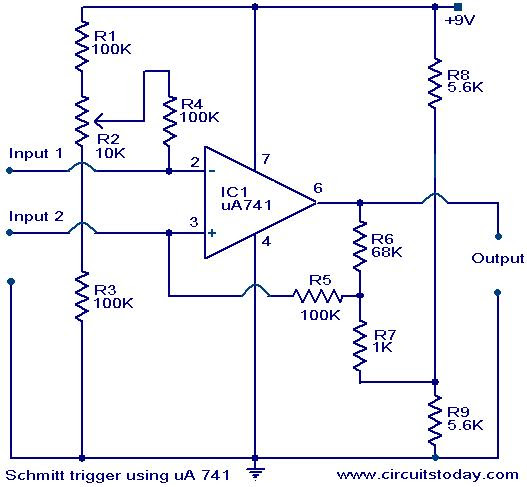## Audio

[Audio][slideshow]

Description.

A Schmitt trigger circuit shows two distinct signal input levels for turning the circuit ON and OFF. The difference between the Von and Voff voltages called hysteresis Schmitt triggers are useful in converting slowly rising waveforms into fast rising ones and in relay like application.

The circuit shown here uses a 741 with positive feedback via R5,R6 and R7 for fast switching.Voltage dividers R8 and R9 set the DC input voltage to the non-inverting input terminal to half the supply voltage. The amount of positive feedback depends on the ratio of R6 and R7, the larger the ratio,the smaller will be the hysterisis shown by the circuit.Potentiometer R2 sets the DC voltage to the inverting input terminal and thus sets the threshold voltage at which the signal will trigger the circuit. The input signal can be applied to either of the two input terminals.

Circuit diagram with parts List .Notes.

• The circuit can be assembled on good quality PCB or common board.
• The threshold can be set by varying the POT R2.
• The circuit can be powered from a 9V battery or 9V DC power supply.
• It is possible to raise the power supply up to 24V .
• IC1 must be mounted on an IC base.

[Lights][grids]